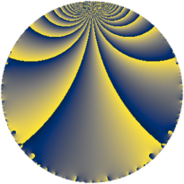Properties

 Label 3640.2.a.lLevel $3640$ Weight $2$ Character orbit 3640.a Self dual yes Analytic conductor $29.066$ Analytic rank $1$ Dimension $2$ CM no Inner twists $1$

Related objects

Newspace parameters

 Level: $$N$$ $$=$$ $$3640 = 2^{3} \cdot 5 \cdot 7 \cdot 13$$ Weight: $$k$$ $$=$$ $$2$$ Character orbit: $$[\chi]$$ $$=$$ 3640.a (trivial)

Newform invariants

 Self dual: yes Analytic conductor: $$29.0655463357$$ Analytic rank: $$1$$ Dimension: $$2$$ Coefficient field: $$\Q(\sqrt{13})$$ Defining polynomial: $$x^{2} - x - 3$$ Coefficient ring: $$\Z[a_1, a_2, a_3]$$ Coefficient ring index: $$1$$ Twist minimal: yes Fricke sign: $$1$$ Sato-Tate group: $\mathrm{SU}(2)$

$q$-expansion

Coefficients of the $$q$$-expansion are expressed in terms of $$\beta = \frac{1}{2}(1 + \sqrt{13})$$. We also show the integral $$q$$-expansion of the trace form.

 $$f(q)$$ $$=$$ $$q -\beta q^{3} + q^{5} + q^{7} + \beta q^{9} +O(q^{10})$$ $$q -\beta q^{3} + q^{5} + q^{7} + \beta q^{9} + ( -2 + 2 \beta ) q^{11} - q^{13} -\beta q^{15} + ( 5 - \beta ) q^{17} + ( -5 + \beta ) q^{19} -\beta q^{21} -8 q^{23} + q^{25} + ( -3 + 2 \beta ) q^{27} + ( -2 - 3 \beta ) q^{29} + ( -2 - \beta ) q^{31} -6 q^{33} + q^{35} + 3 \beta q^{37} + \beta q^{39} + ( -3 + \beta ) q^{41} -2 q^{43} + \beta q^{45} + ( -2 + 2 \beta ) q^{47} + q^{49} + ( 3 - 4 \beta ) q^{51} + 2 \beta q^{53} + ( -2 + 2 \beta ) q^{55} + ( -3 + 4 \beta ) q^{57} + ( 2 - 7 \beta ) q^{59} + ( 4 + 4 \beta ) q^{61} + \beta q^{63} - q^{65} + ( 5 - 3 \beta ) q^{67} + 8 \beta q^{69} + 2 \beta q^{71} + ( -6 + 2 \beta ) q^{73} -\beta q^{75} + ( -2 + 2 \beta ) q^{77} + ( -3 - 5 \beta ) q^{79} + ( -6 - 2 \beta ) q^{81} + ( -4 + 6 \beta ) q^{83} + ( 5 - \beta ) q^{85} + ( 9 + 5 \beta ) q^{87} + ( -6 + \beta ) q^{89} - q^{91} + ( 3 + 3 \beta ) q^{93} + ( -5 + \beta ) q^{95} + ( -8 + 4 \beta ) q^{97} + 6 q^{99} +O(q^{100})$$ $$\operatorname{Tr}(f)(q)$$ $$=$$ $$2 q - q^{3} + 2 q^{5} + 2 q^{7} + q^{9} + O(q^{10})$$ $$2 q - q^{3} + 2 q^{5} + 2 q^{7} + q^{9} - 2 q^{11} - 2 q^{13} - q^{15} + 9 q^{17} - 9 q^{19} - q^{21} - 16 q^{23} + 2 q^{25} - 4 q^{27} - 7 q^{29} - 5 q^{31} - 12 q^{33} + 2 q^{35} + 3 q^{37} + q^{39} - 5 q^{41} - 4 q^{43} + q^{45} - 2 q^{47} + 2 q^{49} + 2 q^{51} + 2 q^{53} - 2 q^{55} - 2 q^{57} - 3 q^{59} + 12 q^{61} + q^{63} - 2 q^{65} + 7 q^{67} + 8 q^{69} + 2 q^{71} - 10 q^{73} - q^{75} - 2 q^{77} - 11 q^{79} - 14 q^{81} - 2 q^{83} + 9 q^{85} + 23 q^{87} - 11 q^{89} - 2 q^{91} + 9 q^{93} - 9 q^{95} - 12 q^{97} + 12 q^{99} + O(q^{100})$$

Embeddings

For each embedding $$\iota_m$$ of the coefficient field, the values $$\iota_m(a_n)$$ are shown below.

For more information on an embedded modular form you can click on its label.

Label $$\iota_m(\nu)$$ $$a_{2}$$ $$a_{3}$$ $$a_{4}$$ $$a_{5}$$ $$a_{6}$$ $$a_{7}$$ $$a_{8}$$ $$a_{9}$$ $$a_{10}$$
1.1
 2.30278 −1.30278
0 −2.30278 0 1.00000 0 1.00000 0 2.30278 0
1.2 0 1.30278 0 1.00000 0 1.00000 0 −1.30278 0
 $$n$$: e.g. 2-40 or 990-1000 Significant digits: Format: Complex embeddings Normalized embeddings Satake parameters Satake angles

Atkin-Lehner signs

$$p$$ Sign
$$2$$ $$1$$
$$5$$ $$-1$$
$$7$$ $$-1$$
$$13$$ $$1$$

Inner twists

This newform does not admit any (nontrivial) inner twists.

Twists

By twisting character orbit
Char Parity Ord Mult Type Twist Min Dim
1.a even 1 1 trivial 3640.2.a.l 2
4.b odd 2 1 7280.2.a.bh 2

By twisted newform orbit
Twist Min Dim Char Parity Ord Mult Type
3640.2.a.l 2 1.a even 1 1 trivial
7280.2.a.bh 2 4.b odd 2 1

Hecke kernels

This newform subspace can be constructed as the intersection of the kernels of the following linear operators acting on $$S_{2}^{\mathrm{new}}(\Gamma_0(3640))$$:

 $$T_{3}^{2} + T_{3} - 3$$ $$T_{11}^{2} + 2 T_{11} - 12$$

Hecke characteristic polynomials

$p$ $F_p(T)$
$2$ $$T^{2}$$
$3$ $$-3 + T + T^{2}$$
$5$ $$( -1 + T )^{2}$$
$7$ $$( -1 + T )^{2}$$
$11$ $$-12 + 2 T + T^{2}$$
$13$ $$( 1 + T )^{2}$$
$17$ $$17 - 9 T + T^{2}$$
$19$ $$17 + 9 T + T^{2}$$
$23$ $$( 8 + T )^{2}$$
$29$ $$-17 + 7 T + T^{2}$$
$31$ $$3 + 5 T + T^{2}$$
$37$ $$-27 - 3 T + T^{2}$$
$41$ $$3 + 5 T + T^{2}$$
$43$ $$( 2 + T )^{2}$$
$47$ $$-12 + 2 T + T^{2}$$
$53$ $$-12 - 2 T + T^{2}$$
$59$ $$-157 + 3 T + T^{2}$$
$61$ $$-16 - 12 T + T^{2}$$
$67$ $$-17 - 7 T + T^{2}$$
$71$ $$-12 - 2 T + T^{2}$$
$73$ $$12 + 10 T + T^{2}$$
$79$ $$-51 + 11 T + T^{2}$$
$83$ $$-116 + 2 T + T^{2}$$
$89$ $$27 + 11 T + T^{2}$$
$97$ $$-16 + 12 T + T^{2}$$